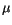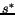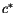Computational & Technology Resources an online resource for computational,engineering & technology publications not logged in - login Computational Science, Engineering & Technology SeriesISSN 1759-3158 CSETS: 10PROGRESS IN CIVIL AND STRUCTURAL ENGINEERING COMPUTING Edited by: B.H.V. Topping Chapter 7Stochastic Optimization Methods in Plastic Analysis and Optimal Plastic Design K. MartiAero-Space Engineering and Technology, Federal Armed Forces University Munich, Neubiberg/Munich, Germany doi:10.4203/csets.10.7 Full Bibliographic Reference for this chapter K. Marti, "Stochastic Optimization Methods in Plastic Analysis and Optimal Plastic Design", in B.H.V. Topping, (Editor), "Progress in Civil and Structural Engineering Computing", Saxe-Coburg Publications, Stirlingshire, UK, Chapter 7, pp 171-189, 2003. doi:10.4203/csets.10.7 Keywords: plastic analysis, optimal plastic design, optimal design under stochastic uncertainty, robust optimal design, stochastic optimization methods, stochastic linear programming. Summary Introduction In plastic analysis and optimal plastic design of elastoplastic mechanical structures one has  to optimize a certain performance function (load factor, weight, initial costs, etc.) under a safety condition consisting of the equilibrium equation and the yield or strength condition. Moreover, there are always some additional simple deterministic constraints, such as box constraints. A basic problem is that due to random material variations, random variations of the loadings, manufacturing and modelling errors, the material resistance or strength parameters of the structure, as e.g. the yield stresses, plastic capacities, etc., as well as the external loads and certain cost factors, are not given, fixed quantities, but random variables having a certain probability distribution. In order to omit a structural weakness and therefore a possible failure or severe damage and then very expensive repair or reconstruction actions, the available a priori information about the random variations of the unknown technical parameters and loadings should be taken into account from the beginning. Hence, in order to get robust optimal solutions with respect to random parameter variations, the original limit analysis/shakedown analysis or optimal design problem with random parameters must be replaced by an appropriate deterministic substitute problem [2,3]. Limit state functions Starting from the structural safety conditions, i.e., the convex or linear yield/strength condition and the linear equilibrium equation involving the member forces and bending moments or the generic stress vectors in the chosen reference points of the structure, based on the static theorem and the kinematic theorem, which are equivalent according to Lagrange duality theory, reliability-based plastic analysis and optimal design problems minimizing e.g. the total expected costs including the expected costs of failure can be described in the framework of a two-stage stochastic (linear) program (SLP) "with complete fixed recourse", having a special staircase structure. For finding this representation, the structural failure is evaluated first by a scalar functionwhich is the minimum value function of a convex or linear program (LP) based on the static/kinematic theorem of the structure. This limit state function can be interpreted as the resistance reserve (positive or negative) of the structure based on the present layout. As an important basic property of, the affine-linearity ofwith respect to the load factoris shown . Then, the corresponding cost functionfor the cost evaluation of inadmissible stress states can be represented by the minimum value function of a certain convex or linear program. Appropriate cost factors in the cost/loss functions are obtained, e.g. for trusses or frames, by taking into account the elastic/plastic strains in the elements of the structure caused by the member forces or bending moments of the structure. Computation of robust optimal designs/load factors For the computation of robust optimal solutions, we consider the following basic deterministic substitute problems: i) Maximize the expected initial costs subject to expected recourse cost constraints and ii) Minimize the expected total costs (initial plus recourse costs). In case of piecewise linear, convex cost functions, robust optimal solutions can be determined numerically by solving a stochastic linear program with complete fixed recourse having the following properties: a) Convexity: The resulting substitute problem is convex for arbitrary probability distributions of the random parameters; b) LP-structure: In case of discrete probability distributions and after piecewise linearization of the convex yield condition, the resulting substitute problem can be represented by a (large scale) deterministic linear program with a "dual block angular data structure"; c) LP-solvers: For solving LP's of this type, several special purpose LP-solvers are available exploiting the special structure of the linear program. d) Arbitrary probability distributions: For an arbitrary (continuous) probability distribution of the random parameters, the resulting substitute problems can be solved approximatively by means of a discretization of the given probability distribution and using then the above mentioned SLP-methods. Convergence results are available. References 1 K. Marti, "Approximation and derivatives of probabilities of survival in structural analysis and design", Structural Optimization, 13, 230-243, 1997. doi:10.1007/BF01197451 2 K. Marti, "Optimal structural design under stochastic uncertainty by stochastic linear programming methods", Reliability Engineering and System Safety (RESS), 72 (2), 165-177, 2001. doi:10.1016/S0951-8320(01)00003-5 3 K. Marti, "Plastic Structural Analysis under Stochastic Uncertainty", To appear in MCMDS, 2003. doi:10.1076/mcmd.9.3.303.24149 purchase the full-text of this chapter (price £20) Back to top ©Civil-Comp Limited 2020 - terms & conditions